# RD Sharma Solutions for Class 8 Maths Chapter 4 - Cubes and Cube Roots Exercise 4.3

Download free pdf of RD Sharma Class 8 Maths from the links provided below. Students can refer to RD Sharma textbook and then practice the RD Sharma Class 8 Solutions provided by BYJU’S expert team. The solutions are solved using simple techniques for easy understanding, which help in achieving high marks in their examination. In Exercise 4.3 of RD Sharma Solutions for Class 8 Maths Chapter 4 Cubes and Cube Roots, we shall discuss problems based on cube roots and cube root of a natural number using units digit method, cube root of a perfect cube by factors.

## Download PDF of RD Sharma Solutions for Class 8 Maths Exercise 4.3 Chapter 4 Cubes and Cube Roots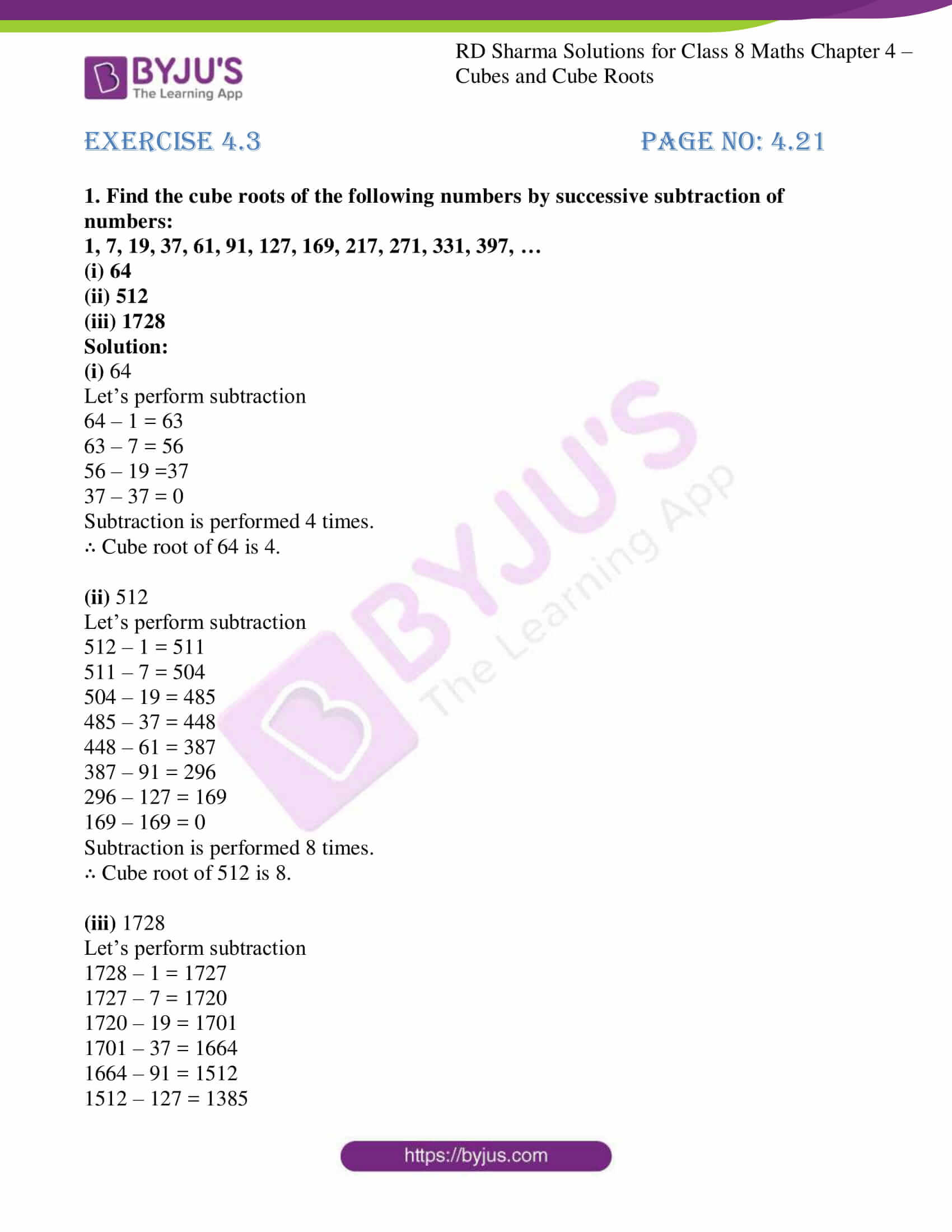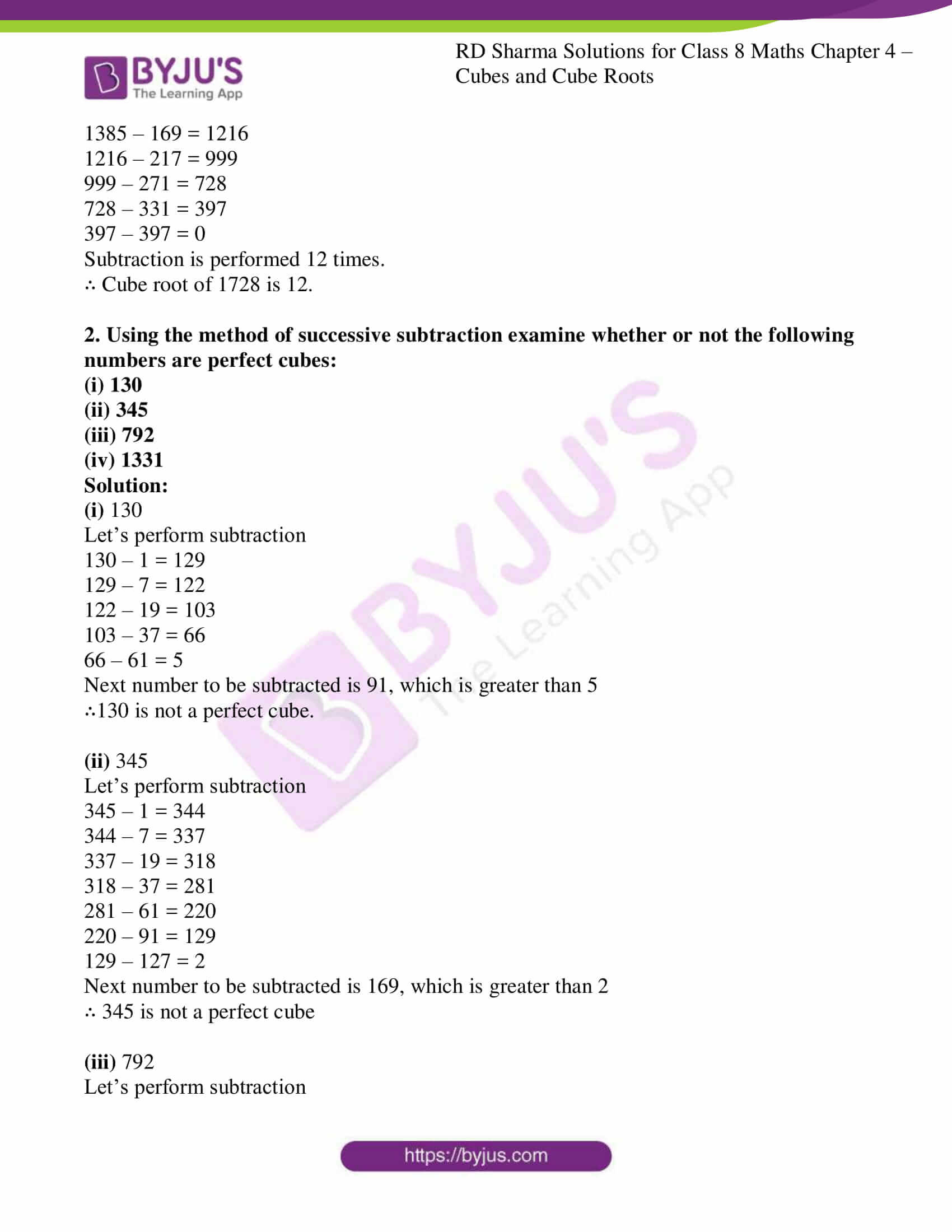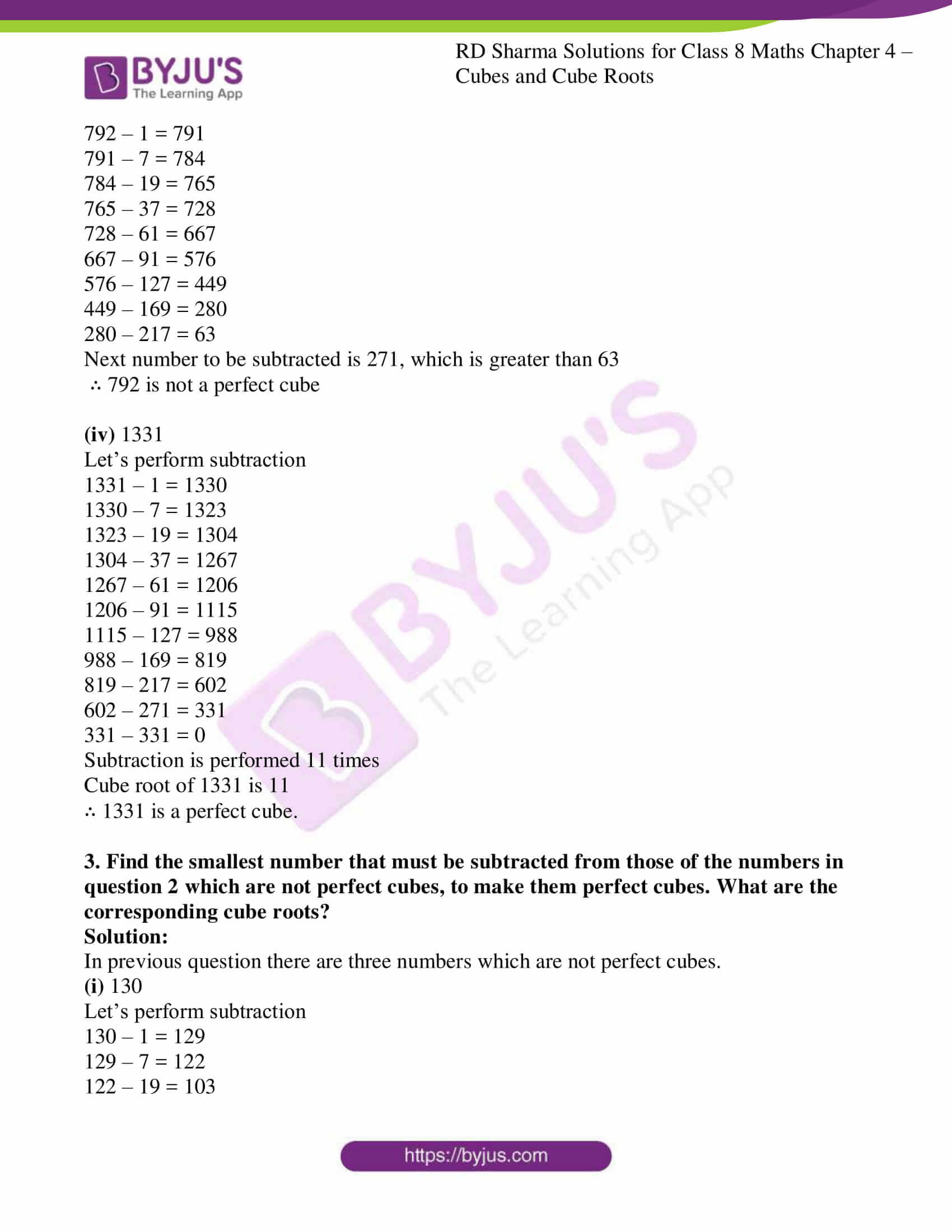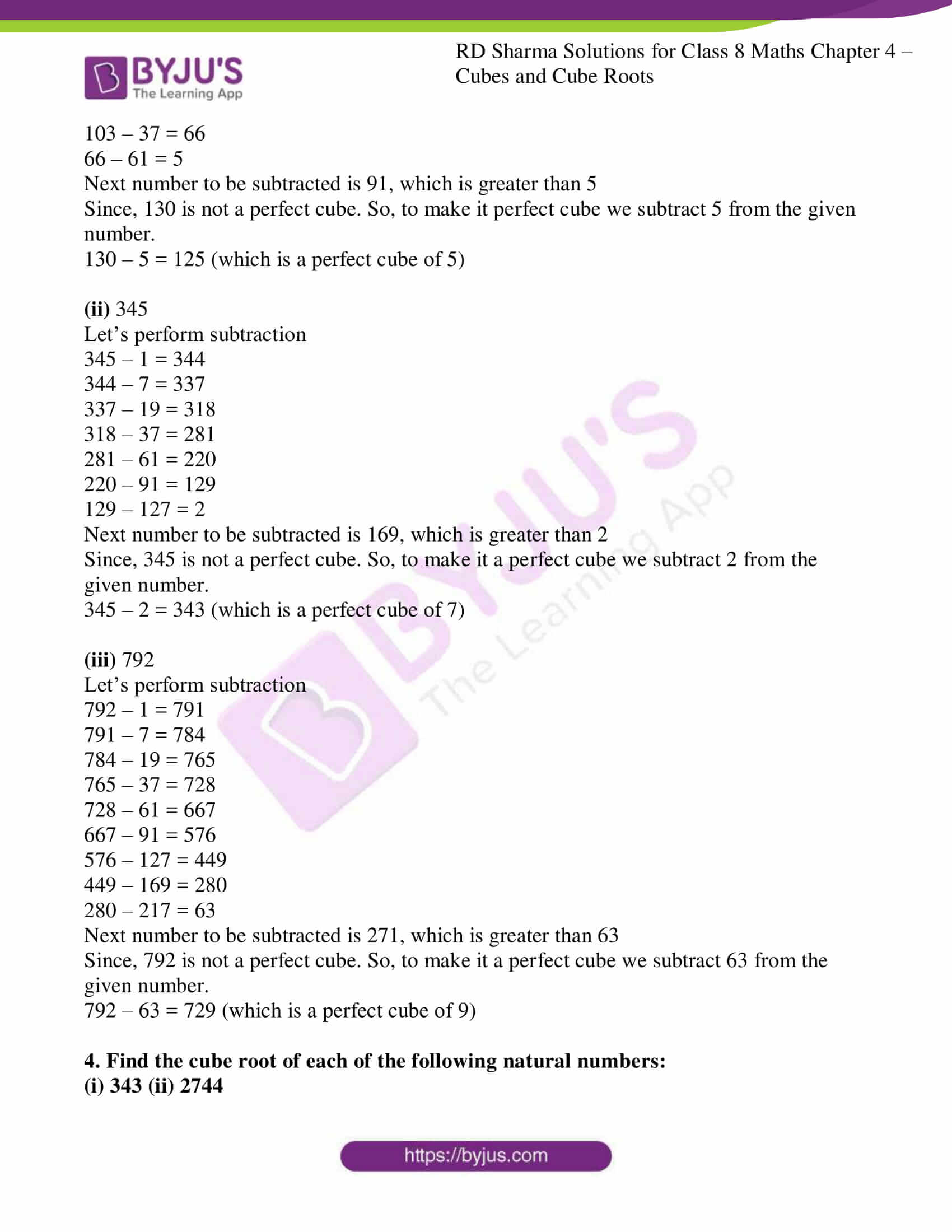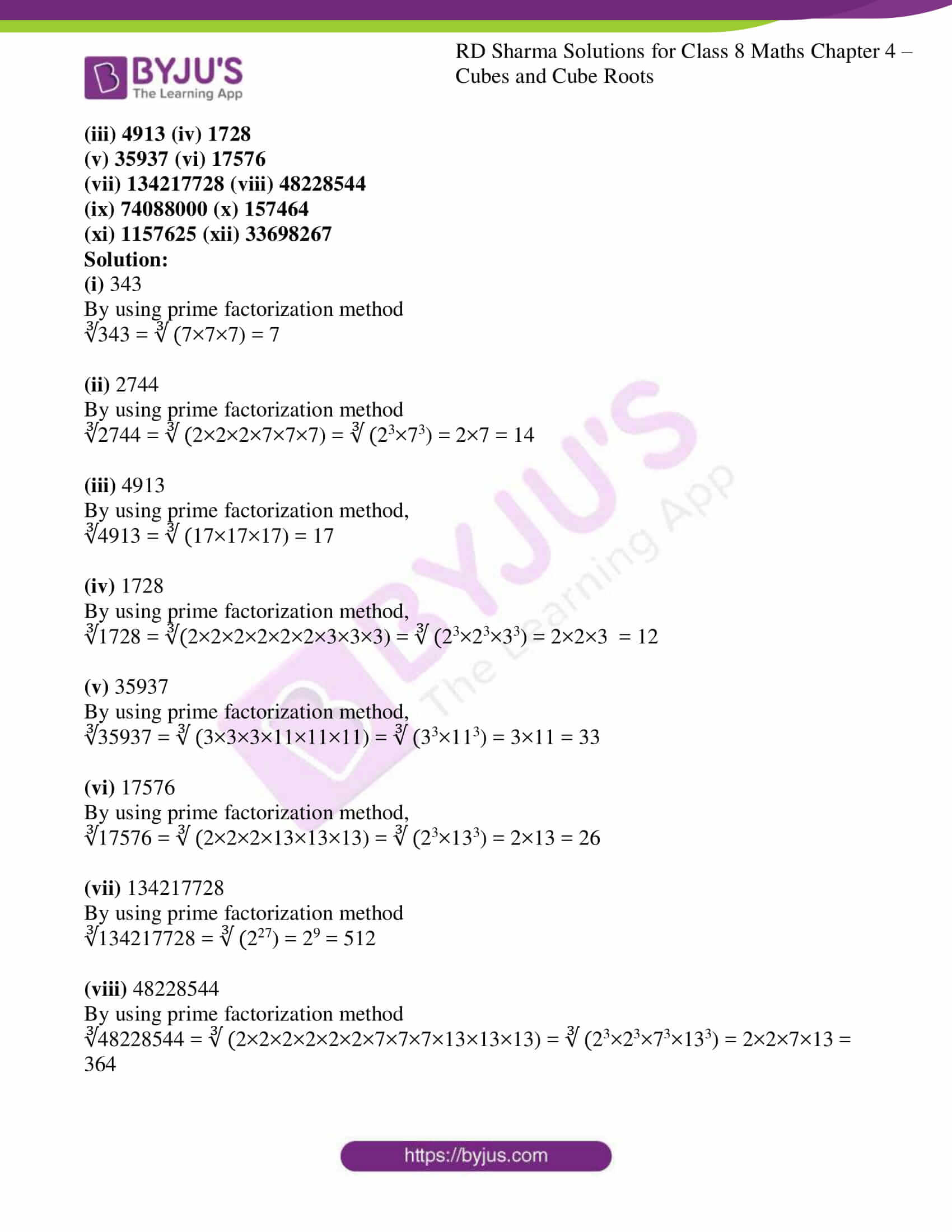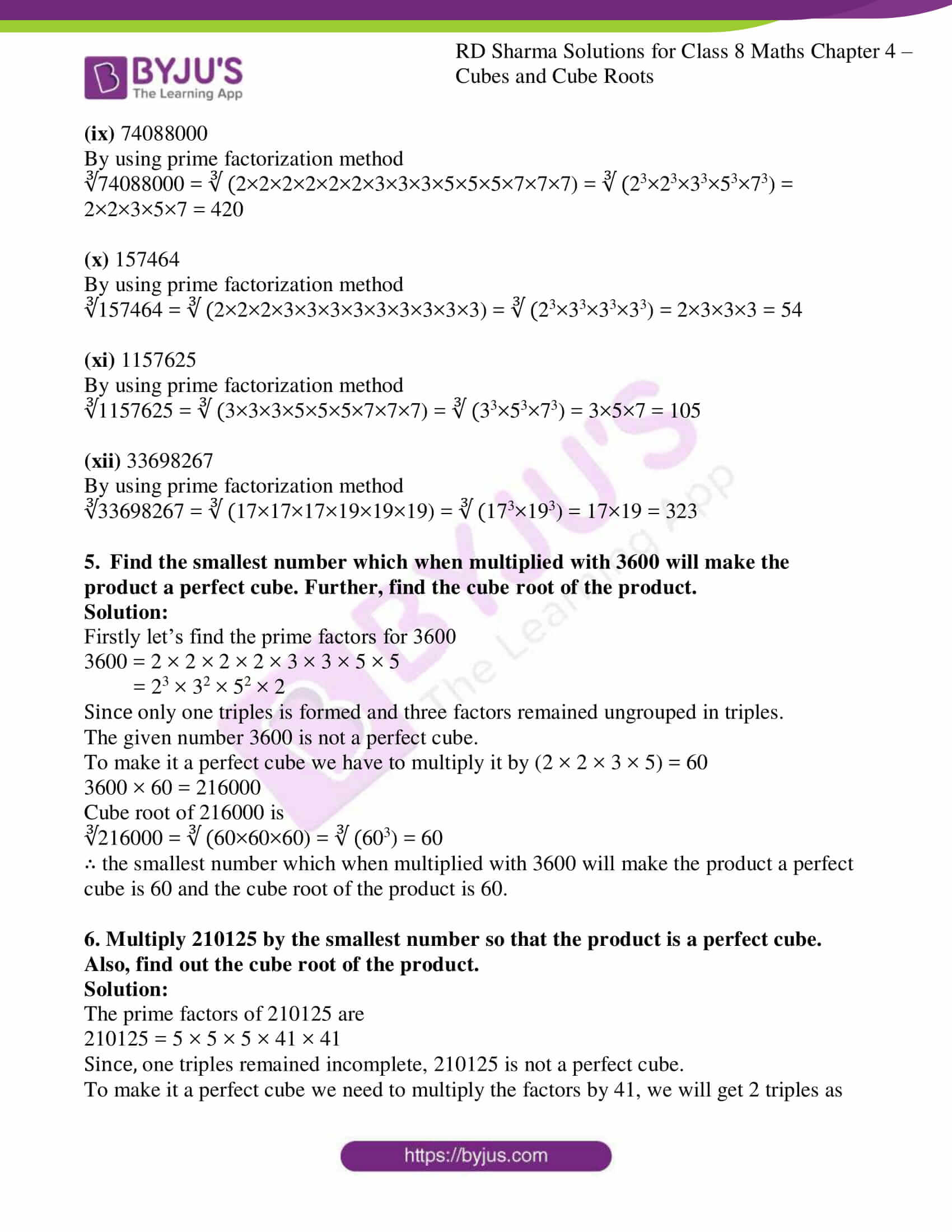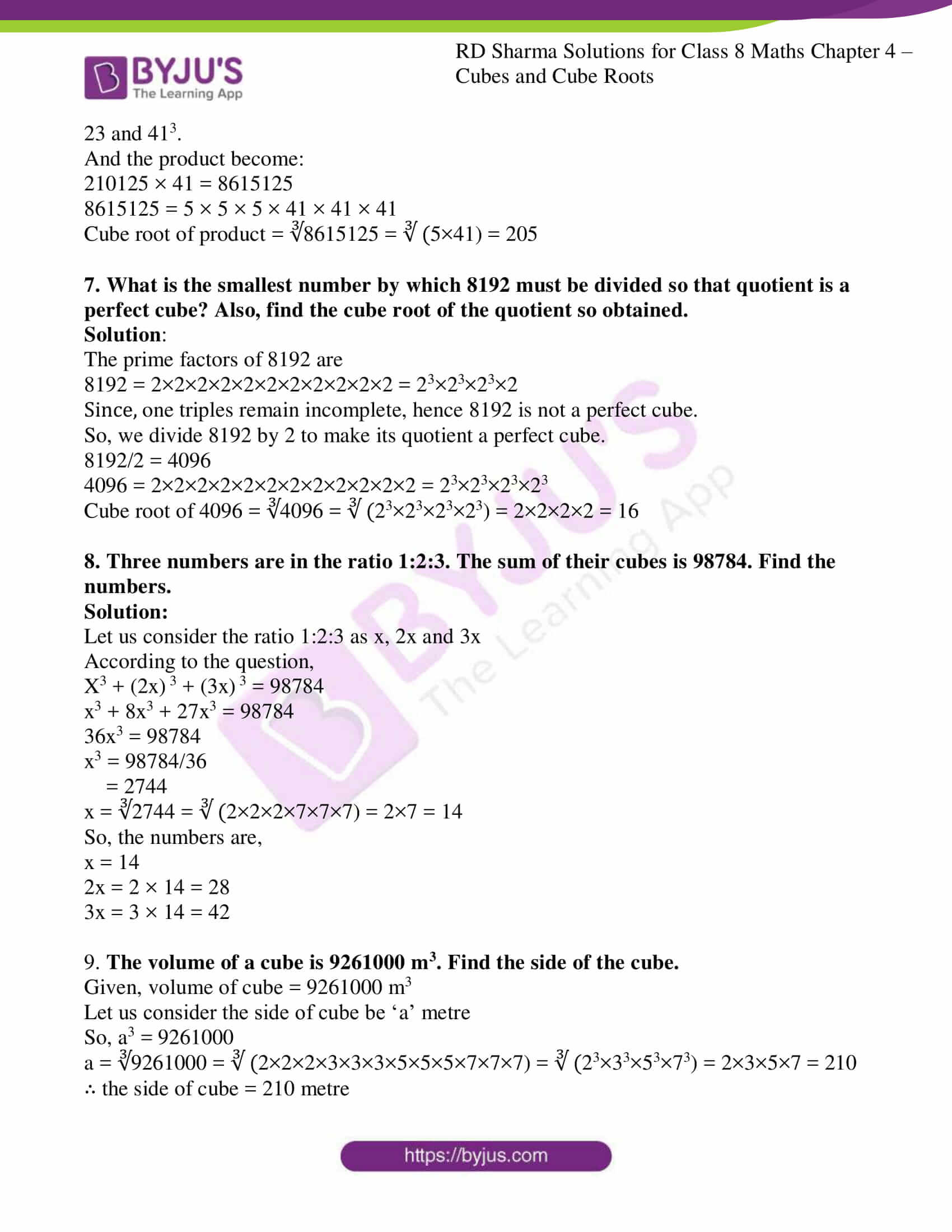### Access Answers to RD Sharma Solutions for Class 8 Maths Exercise 4.3 Chapter 4 Cubes and Cube Roots

1. Find the cube roots of the following numbers by successive subtraction of numbers:
1, 7, 19, 37, 61, 91, 127, 169, 217, 271, 331, 397, …
(i) 64
(ii) 512
(iii) 1728

Solution:

(i) 64

Let’s perform subtraction

64 – 1 = 63

63 – 7 = 56

56 – 19 =37

37 – 37 = 0

Subtraction is performed 4 times.

∴ Cube root of 64 is 4.

(ii) 512

Let’s perform subtraction

512 – 1 = 511

511 – 7 = 504

504 – 19 = 485

485 – 37 = 448

448 – 61 = 387

387 – 91 = 296

296 – 127 = 169

169 – 169 = 0

Subtraction is performed 8 times.

∴ Cube root of 512 is 8.

(iii) 1728

Let’s perform subtraction

1728 – 1 = 1727

1727 – 7 = 1720

1720 – 19 = 1701

1701 – 37 = 1664

1664 – 91 = 1512

1512 – 127 = 1385

1385 – 169 = 1216

1216 – 217 = 999

999 – 271 = 728

728 – 331 = 397

397 – 397 = 0

Subtraction is performed 12 times.

∴ Cube root of 1728 is 12.

2. Using the method of successive subtraction examine whether or not the following numbers are perfect cubes:
(i) 130
(ii) 345
(iii) 792
(iv) 1331

Solution:

(i) 130

Let’s perform subtraction

130 – 1 = 129

129 – 7 = 122

122 – 19 = 103

103 – 37 = 66

66 – 61 = 5

Next number to be subtracted is 91, which is greater than 5

∴130 is not a perfect cube.

(ii) 345

Let’s perform subtraction

345 – 1 = 344

344 – 7 = 337

337 – 19 = 318

318 – 37 = 281

281 – 61 = 220

220 – 91 = 129

129 – 127 = 2

Next number to be subtracted is 169, which is greater than 2

∴ 345 is not a perfect cube

(iii) 792

Let’s perform subtraction

792 – 1 = 791

791 – 7 = 784

784 – 19 = 765

765 – 37 = 728

728 – 61 = 667

667 – 91 = 576

576 – 127 = 449

449 – 169 = 280

280 – 217 = 63

Next number to be subtracted is 271, which is greater than 63

∴ 792 is not a perfect cube

(iv) 1331

Let’s perform subtraction

1331 – 1 = 1330

1330 – 7 = 1323

1323 – 19 = 1304

1304 – 37 = 1267

1267 – 61 = 1206

1206 – 91 = 1115

1115 – 127 = 988

988 – 169 = 819

819 – 217 = 602

602 – 271 = 331

331 – 331 = 0

Subtraction is performed 11 times

Cube root of 1331 is 11

∴ 1331 is a perfect cube.

3. Find the smallest number that must be subtracted from those of the numbers in question 2 which are not perfect cubes, to make them perfect cubes. What are the corresponding cube roots?

Solution:

In previous question there are three numbers which are not perfect cubes.

(i) 130

Let’s perform subtraction

130 – 1 = 129

129 – 7 = 122

122 – 19 = 103

103 – 37 = 66

66 – 61 = 5

Next number to be subtracted is 91, which is greater than 5

Since, 130 is not a perfect cube. So, to make it perfect cube we subtract 5 from the given number.

130 – 5 = 125 (which is a perfect cube of 5)

(ii) 345

Let’s perform subtraction

345 – 1 = 344

344 – 7 = 337

337 – 19 = 318

318 – 37 = 281

281 – 61 = 220

220 – 91 = 129

129 – 127 = 2

Next number to be subtracted is 169, which is greater than 2

Since, 345 is not a perfect cube. So, to make it a perfect cube we subtract 2 from the given number.

345 – 2 = 343 (which is a perfect cube of 7)

(iii) 792

Let’s perform subtraction

792 – 1 = 791

791 – 7 = 784

784 – 19 = 765

765 – 37 = 728

728 – 61 = 667

667 – 91 = 576

576 – 127 = 449

449 – 169 = 280

280 – 217 = 63

Next number to be subtracted is 271, which is greater than 63

Since, 792 is not a perfect cube. So, to make it a perfect cube we subtract 63 from the given number.

792 – 63 = 729 (which is a perfect cube of 9)

4. Find the cube root of each of the following natural numbers:
(i) 343 (ii) 2744

(iii) 4913 (iv) 1728
(v) 35937 (vi) 17576
(vii) 134217728 (viii) 48228544
(ix) 74088000 (x) 157464
(xi) 1157625 (xii) 33698267

Solution:

(i) 343

By using prime factorization method

∛343 = ∛ (7×7×7) = 7

(ii) 2744

By using prime factorization method

∛2744 = ∛ (2×2×2×7×7×7) = ∛ (23×73) = 2×7 = 14

(iii) 4913

By using prime factorization method,

∛4913 = ∛ (17×17×17) = 17

(iv) 1728

By using prime factorization method,

∛1728 = ∛(2×2×2×2×2×2×3×3×3) = ∛ (23×23×33) = 2×2×3 = 12

(v) 35937

By using prime factorization method,

∛35937 = ∛ (3×3×3×11×11×11) = ∛ (33×113) = 3×11 = 33

(vi) 17576

By using prime factorization method,

∛17576 = ∛ (2×2×2×13×13×13) = ∛ (23×133) = 2×13 = 26

(vii) 134217728

By using prime factorization method

∛134217728 = ∛ (227) = 29 = 512

(viii) 48228544

By using prime factorization method

∛48228544 = ∛ (2×2×2×2×2×2×7×7×7×13×13×13) = ∛ (23×23×73×133) = 2×2×7×13 = 364

(ix) 74088000

By using prime factorization method

∛74088000 = ∛ (2×2×2×2×2×2×3×3×3×5×5×5×7×7×7) = ∛ (23×23×33×53×73) = 2×2×3×5×7 = 420

(x) 157464

By using prime factorization method

∛157464 = ∛ (2×2×2×3×3×3×3×3×3×3×3×3) = ∛ (23×33×33×33) = 2×3×3×3 = 54

(xi) 1157625

By using prime factorization method

∛1157625 = ∛ (3×3×3×5×5×5×7×7×7) = ∛ (33×53×73) = 3×5×7 = 105

(xii) 33698267

By using prime factorization method

∛33698267 = ∛ (17×17×17×19×19×19) = ∛ (173×193) = 17×19 = 323

5. Find the smallest number which when multiplied with 3600 will make the product a perfect cube. Further, find the cube root of the product.

Solution:

Firstly let’s find the prime factors for 3600

3600 = 2 × 2 × 2 × 2 × 3 × 3 × 5 × 5

= 23 × 32 × 52 × 2

Since only one triples is formed and three factors remained ungrouped in triples.

The given number 3600 is not a perfect cube.

To make it a perfect cube we have to multiply it by (2 × 2 × 3 × 5) = 60

3600 × 60 = 216000

Cube root of 216000 is

∛216000 = ∛ (60×60×60) = ∛ (603) = 60

∴ the smallest number which when multiplied with 3600 will make the product a perfect cube is 60 and the cube root of the product is 60.

6. Multiply 210125 by the smallest number so that the product is a perfect cube. Also, find out the cube root of the product.

Solution:

The prime factors of 210125 are

210125 = 5 × 5 × 5 × 41 × 41

Since, one triples remained incomplete, 210125 is not a perfect cube.

To make it a perfect cube we need to multiply the factors by 41, we will get 2 triples as 23 and 413.

And the product become:

210125 × 41 = 8615125

8615125 = 5 × 5 × 5 × 41 × 41 × 41

Cube root of product = ∛8615125 = ∛ (5×41) = 205

7. What is the smallest number by which 8192 must be divided so that quotient is a perfect cube? Also, find the cube root of the quotient so obtained.

Solution:

The prime factors of 8192 are

8192 = 2×2×2×2×2×2×2×2×2×2×2 = 23×23×23×2

Since, one triples remain incomplete, hence 8192 is not a perfect cube.

So, we divide 8192 by 2 to make its quotient a perfect cube.

8192/2 = 4096

4096 = 2×2×2×2×2×2×2×2×2×2×2×2 = 23×23×23×23

Cube root of 4096 = ∛4096 = ∛ (23×23×23×23) = 2×2×2×2 = 16

8. Three numbers are in the ratio 1:2:3. The sum of their cubes is 98784. Find the numbers.

Solution:

Let us consider the ratio 1:2:3 as x, 2x and 3x

According to the question,

X3 + (2x) 3 + (3x) 3 = 98784

x3 + 8x3 + 27x3 = 98784

36x3 = 98784

x3 = 98784/36

= 2744

x = ∛2744 = ∛ (2×2×2×7×7×7) = 2×7 = 14

So, the numbers are,

x = 14

2x = 2 × 14 = 28

3x = 3 × 14 = 42

9. The volume of a cube is 9261000 m3. Find the side of the cube.

Given, volume of cube = 9261000 m3

Let us consider the side of cube be ‘a’ metre

So, a3 = 9261000

a = ∛9261000 = ∛ (2×2×2×3×3×3×5×5×5×7×7×7) = ∛ (23×33×53×73) = 2×3×5×7 = 210

∴ the side of cube = 210 metre